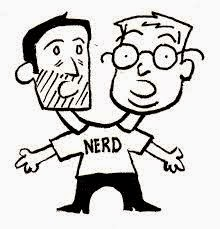# A Special Old GuyAlvin and Buzz are nerds and like doing nerdy things. So Alvin called Buzz one day...

"Buzz, I've finished tracing my family tree back from the year 500 AD, and I found one quite special guy".

"Well, he was x years old in the year x^2 (x squared) and he had a son who was y years old in the year y^3 (y cubed)".

Buzz looked perplexed "Sorry Alvin, but I can't solve for x or y".

"Well, he was your age when his son was born." said Alvin.

"You're right" said Buzz "He was a special old guy! But I still can't solve for x or y".

How old was the old guy when his son was born?

Notes:
Assume that the nerds have the conversation this year, ie 2004 AD.

### A Special Old Guy Puzzle Solution

Firstly, we can say that the date of birth (DOB) for the special old guy (SOG) is:
Equation {a}:
DOB(SOG) = x^2 – x

Similarly, for the son of the special old guy (or SOSOG for short):

Equation {b}:
DOB(SOSOG) = y^3 – y

SOG's age when SOSOG was born was:
Equation {c}:
Age(SOG) = DOB(SOSOG) – DOB(SOG) = (y^3 – y) – (x^2 – x)

There are unlimited solutions to equation {c} so we need some assumptions and limits.

We know that SOG must have been between, say 10 years old and 100 years old when SOSOG was born:

Equation {d}:
10 < Age(SOG) < 100

We also know that both SOG and SOSOG were born some time since the year 500 AD:
Equation {e}:
500 < DOB(SOSOG) < 2004.

Based on equation {b} and {e} we can clearly see that there are only 5 solutions for y. They are y = 8, 9, 10, 11 or 12. Any other solutions for y are in breach of equation {e}.
For each of these possible solutions for y there is only a limited number of solutions for x that comply with {d}. They are:
``` x     y
--------
21     8
22     8
26     9
27     9
31    10
36    11
41    12
```

Any other solutions for x and y are in breach of equation {d}.
SOG's age when his son was born can be calculated for each of these possible solutions by using equation {c} as follows:
``` x      y     Age(SOG)
----------------------
21      8           84
22      8           42
26      9           70
27      9           18
31     10           60
36     11           60
41     12           76
```

So there are 7 different solutions, which is why Buzz said he couldn't solve for x and y.
Now comes the lateral part of the puzzle: Alvin informs Buzz that "...he was your age when his son was born". Of course, Buzz knows his own age. He should therefore be able to pick the correct solution from the list of 7 possible solutions shown above. However, he can't. That means that Buzz (and the special old guy) must be 60 because there are multiple solutions for an age of 60, whereas any other age would yield a unique solution. Any other age and Buzz would be able to solve.
A 60 year old father – quite a special old guy.

#### 1 comment :

1.Sebagai dadu online terpercaya kami selalu memberikan pelayanan terbaik bagi member.